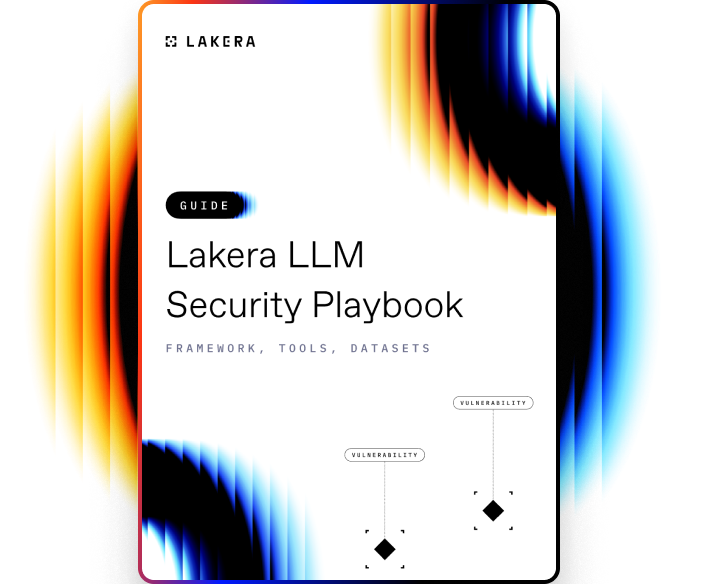VOCABULARY

# Cross-Validation Modeling

Cross-validation modeling is a resampling procedure used to evaluate machine learning models on a limited data sample. It is a statistical method that is primarily used to estimate the skill of machine learning models. Cross-validation is intended to prevent overfitting, which refers to the scenario where the model fits perfectly on the training data but performs poorly on new, unseen data.

## How Cross-validation Modeling works

Cross-validation works by splitting the dataset into two segments: one used to train a model and the other used to validate the model. The most common method is the k-fold cross-validation where the original sample is randomly partitioned into k equal-sized subsamples. Of the k subsamples, a single subsample is retained as the validation data for testing the model, and the remaining k-1 subsamples are used as training data.

The process is then repeated k times (the folds), with each of the k subsamples used exactly once as the validation data. The k results from the folds can then be averaged (or otherwise combined) to produce a single estimation. This method allows for every observation from the original sample to appear in the training and test set. It is particularly useful when the prediction error needs to be minimized.Learn how to protect against the most common LLM vulnerabilities

Download this guide to delve into the most common LLM security risks and ways to mitigate them.

Related termsActivate
untouchable mode.